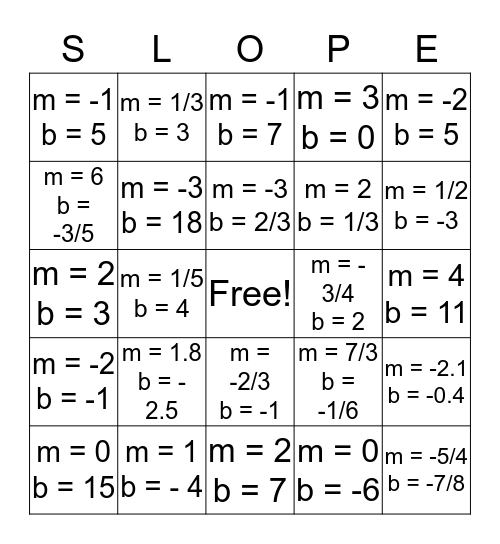# GEOMETRIC SKI RESORTThis bingo card has a free space and 24 words: m = 1.8 b = - 2.5, m = 1 b = - 4, m = -3 b = 2/3, m = 0 b = 15, m = -2 b = 5, m = -1 b = 5, m = 6 b = -3/5, m = 1/3 b = 3, m = - 3/4 b = 2, m = -2/3 b = -1, m = 2 b = 1/3, m = 2 b = 3, m = 7/3 b = -1/6, m = -2.1 b = -0.4, m = -3 b = 18, m = 0 b = -6, m = 4 b = 11, m = -1 b = 7, m = 1/2 b = -3, m = 1/5 b = 4, m = -5/4 b = -7/8, m = -2 b = -1, m = 3 b = 0 and m = 2 b = 7.

## Play Online